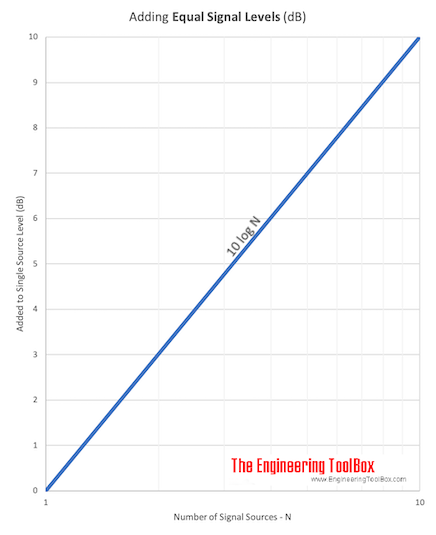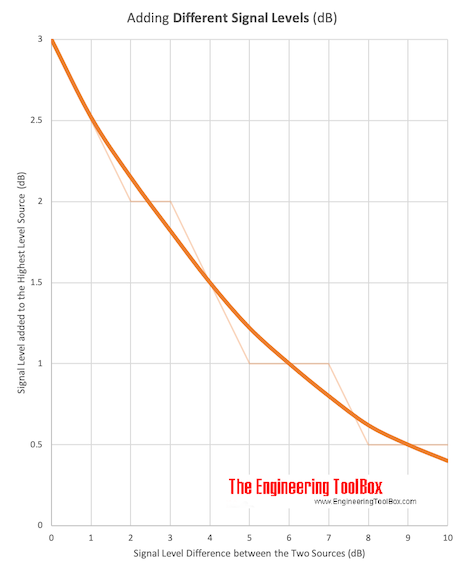Engineering ToolBox - Resources, Tools and Basic Information for Engineering and Design of Technical Applications!

## The logarithmic decibel scale is convenient when adding signal values like sound power, pressure and others from two or more sources.

The decibel (dB) is a logarithmic unit used to express the ratio of two signal values - like power, sound power or pressure, voltage, intensity etc. - where one value is a reference value.

The total signal level in decibel from equal signal sources can be calculated as

Lt =  10 log (n S / Sref)

= 10 log (S / Sref) + 10 log (n)

= Ls + 10 log (n)                                                         (1)

where

Lt = total signal level (dB)

S = signal (signal unit)

Sref = signal reference (signal unit)

n = number of sources

Ls = signal level from each single source (dB)

The signal units depends on the nature of the signal - W for power, Pa for pressure and so on.

Note! -  adding sound pressure levels.

#### Example - Total Sound Power from Two Identical Fans

For sound power it is common to use 10-12 W as the reference sound power. Total sound power from two identical fans each generating 1 W in noise power can be calculated as

Lt =  10 log (2 (1 W) / (1 10-12 W))

= 123 dB

Sound power and sound power level are often used to specify the noise or sound emitted from technical equipment like fans, pumps or other machines. The "sound" measured with microphones or sensors (meters) are sound pressure.

### Adding Equal Signals Units Calculator

S - signal (signal unit)

Sref - signal reference (signal unit)

n - number of signal sources

### Adding Equal Signal Levels (decibels) Calculator

Ls - signal level from single source (decibel, dB)

n - number of signal sources

Adding equal signal sources can be expressed graphicallyDownload and print Adding Signal Level of Equal Sources chart.

Note! Adding two identical sources (doubling the signal) will increase the total signal level with 3 dB (10 log(2)).

Number of
Sources
Increase in
Sound Power Level
(dB)
2 3
3 4.8
4 6
5 7
10 10
15 11.8
20 13

### Adding Signals from Sources with different Strengths

The total signal level from sources with different strengths can be calculated as

Lt =  10 log ((S1 + S2 ... + Sn) / Sref)                                                (2)

#### Example - Total Sound Power from Two different Fans

The total noise power from two fans - one with sound power 1 W and the other with sound power 0.5 W - can be calculated as

Lt =  10 log (((1 W) + (0.5 W)) / (1 10-12 W))

= 122 dB

Adding two signal sources with different levels can be expressed graphically in decibels asSignal Level Difference
between
two Sources
(dB)
Decibels
Highest Signal Level
(dB)
0 3
1 2.5
2 2
3 2
4 1.5
5 1
6 1
7 1
8 0.5
9 0.5
10 0.5
> 10 0

#### Example - Adding Sound Power in Decibels

The sound power from one of the fans in the example above can be calculated as

Ls1 = 10 log((1 W) / (1 10-12 W))

= 120 dB

The sound power from the other fan can be calculated as

Ls2 = 10 log((0.5 W) / (1 10-12 W))

= 117 dB

The difference in decibel is

Ls1 - Ls2

= (120 dB) - (117 dB)

= 3 dB

From the table or diagram above a difference of 3 dB requires that 2 dB must be added to the highest sound pressure source as

Lt = (120 dB) + (2 dB)

= 122 dB

## Related Topics

• ### Acoustics

Room acoustics and acoustic properties, decibel A, B and C, Noise Rating (NR) curves, sound transmission, sound pressure, sound intensity and sound attenuation.
• ### Measurements and Instrumentation

Measurement and instrumentation strategies.
• ### Noise and Attenuation

Noise is usually defined as unwanted sound - noise, noise generation, silencers and attenuation in HVAC systems.

## Related Documents

• ### Decibel

Logarithmic unit used to describe ratios of signal levels - like power or intensity - to a reference level.
• ### Decibel A, B and C

Sound pressure filters that compensates for the hearing sensed by the human ear.
• ### Logarithms

The rules of logarithms - log10 and loge for numbers ranging 1 to 1000.
• ### Noise Attenuation in Rotary Heat Exchanges

Sound attenuation vs. frequency in rotating heat exchangers.
• ### Noise generated in Air Ducts

Estimate noise generated by air flow in ducts.
• ### Noise generated in Blade Dampers

Sound power noise generated by blade dampers in ventilation systems.
• ### Propagation of Outdoor Sound - Partial Barriers

The transmission of outdoor sound through and around barriers - the Fresnel Number.
• ### Sound Intensity, Power and Pressure Levels

Introduction to decibel, sound power, intensity and pressure.
• ### Sound Power

Sound power from sources like fans, jet engines, cars, humans and more.
• ### Sound Pressure

Sound Pressure is the force of sound on a surface perpendicular to the propagation of sound.

## Engineering ToolBox - SketchUp Extension - Online 3D modeling!

Add standard and customized parametric components - like flange beams, lumbers, piping, stairs and more - to your Sketchup model with the Engineering ToolBox - SketchUp Extension - enabled for use with older versions of the amazing SketchUp Make and the newer "up to date" SketchUp Pro . Add the Engineering ToolBox extension to your SketchUp Make/Pro from the Extension Warehouse !

We don't collect information from our users. More about

## Citation

• The Engineering ToolBox (2003). Signals - Adding Decibels. [online] Available at: https://www.engineeringtoolbox.com/adding-decibel-d_63.html [Accessed Day Month Year].

Modify the access date according your visit.

9.19.12